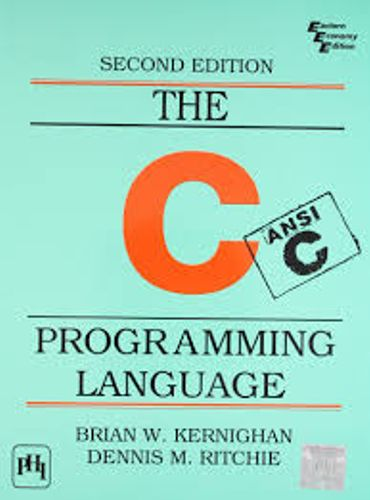# For loops

For loops in C Programming are straightforward. They supply the ability to create a loop – a code block that runs multiple times. For loops require an iterator variable, usually notated as `i`.

For loops give the following functionality:

• Initialize the iterator variable using an initial value
• Check if the iterator has reached its final value
• Increases the iterator

For example, if we wish to iterate on a block for 10 times, we write:

```int i;
for (i = 0; i < 10; i++) {
printf("%d\n", i);
}
```

This block will print the numbers 0 through 9 (10 numbers in total).

For loops can iterate on array values. For example, if we would want to sum all the values of an array, we would use the iterator `i` as the array index:

```int array = { 1, 2, 3, 4, 5, 6, 7, 8, 9, 10 };
int sum = 0;
int i;

for (i = 0; i < 10; i++) {
sum += array[i];
}

/* sum now contains a + a + ... + a */
printf("Sum of the array is %d\n", sum);
```

## Home Work

Calculate the factorial (multiplication of all items `array` to `array`, inclusive), of the variable `array`.

Click on image to Download C Programming Language Book :